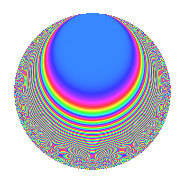# Properties

 Label 349.2.b.bLevel 349 Weight 2 Character orbit 349.b Analytic conductor 2.787 Analytic rank 0 Dimension 26 CM No

# Related objects

## Newspace parameters

 Level: $$N$$ = $$349$$ Weight: $$k$$ = $$2$$ Character orbit: $$[\chi]$$ = 349.b (of order $$2$$ and degree $$1$$)

## Newform invariants

 Self dual: No Analytic conductor: $$2.78677903054$$ Analytic rank: $$0$$ Dimension: $$26$$ Sato-Tate group: $\mathrm{SU}(2)[C_{2}]$

## $q$-expansion

The dimension is sufficiently large that we do not compute an algebraic $$q$$-expansion, but we have computed the trace expansion.

 $$\operatorname{Tr}(f)(q) =$$ $$26q - 2q^{3} - 36q^{4} - 12q^{5} + 32q^{9} + O(q^{10})$$ $$\operatorname{Tr}(f)(q) =$$ $$26q - 2q^{3} - 36q^{4} - 12q^{5} + 32q^{9} + 12q^{12} + 4q^{14} + 12q^{15} + 20q^{16} - 14q^{17} - 4q^{19} - 2q^{20} - 12q^{22} - 18q^{23} + 18q^{25} + 22q^{26} + 4q^{27} - 18q^{29} + 10q^{31} - 54q^{36} + 30q^{37} - 16q^{41} - 44q^{45} - 74q^{48} - 22q^{49} + 32q^{51} - 38q^{56} - 16q^{57} - 78q^{60} - 96q^{64} + 104q^{66} + 72q^{67} + 36q^{68} - 40q^{69} + 86q^{70} + 72q^{73} - 38q^{75} + 96q^{76} - 28q^{77} - 30q^{78} + 30q^{80} - 6q^{81} - 8q^{83} - 22q^{85} + 60q^{86} + 32q^{87} + 110q^{88} - 12q^{91} + 14q^{92} + 84q^{93} + 22q^{94} - 10q^{95} + O(q^{100})$$

## Embeddings

For each embedding $$\iota_m$$ of the coefficient field, the values $$\iota_m(a_n)$$ are shown below.

For more information on an embedded modular form you can click on its label.

Label $$a_{2}$$ $$a_{3}$$ $$a_{4}$$ $$a_{5}$$ $$a_{6}$$ $$a_{7}$$ $$a_{8}$$ $$a_{9}$$ $$a_{10}$$
348.1 2.74676i −1.22099 −5.54469 1.97385 3.35376i 4.26150i 9.73641i −1.50918 5.42170i
348.2 2.61918i −2.83744 −4.86011 −3.82575 7.43177i 2.86678i 7.49115i 5.05107 10.0203i
348.3 2.51815i 2.41625 −4.34106 2.06598 6.08448i 1.34000i 5.89514i 2.83827 5.20243i
348.4 2.26080i 0.674297 −3.11122 −0.0827003 1.52445i 1.39223i 2.51226i −2.54532 0.186969i
348.5 2.07129i −0.0823577 −2.29025 −3.08948 0.170587i 3.12974i 0.601197i −2.99322 6.39921i
348.6 1.88002i −2.43904 −1.53447 2.91531 4.58543i 0.518323i 0.875209i 2.94890 5.48083i
348.7 1.82942i 3.30797 −1.34678 −0.842567 6.05168i 2.41293i 1.19501i 7.94269 1.54141i
348.8 1.36584i 2.13942 0.134484 −3.98594 2.92210i 4.87861i 2.91536i 1.57710 5.44415i
348.9 1.25654i 0.849271 0.421101 3.08880 1.06714i 1.10667i 3.04222i −2.27874 3.88121i
348.10 1.19339i −2.66615 0.575830 −1.00436 3.18175i 3.16046i 3.07396i 4.10838 1.19859i
348.11 0.980996i −2.39151 1.03765 −1.59459 2.34607i 3.49225i 2.97992i 2.71933 1.56429i
348.12 0.788732i −0.670838 1.37790 −1.84585 0.529112i 0.0300147i 2.66426i −2.54998 1.45588i
348.13 0.719982i 1.92112 1.48163 0.227297 1.38317i 2.96485i 2.50671i 0.690697 0.163649i
348.14 0.719982i 1.92112 1.48163 0.227297 1.38317i 2.96485i 2.50671i 0.690697 0.163649i
348.15 0.788732i −0.670838 1.37790 −1.84585 0.529112i 0.0300147i 2.66426i −2.54998 1.45588i
348.16 0.980996i −2.39151 1.03765 −1.59459 2.34607i 3.49225i 2.97992i 2.71933 1.56429i
348.17 1.19339i −2.66615 0.575830 −1.00436 3.18175i 3.16046i 3.07396i 4.10838 1.19859i
348.18 1.25654i 0.849271 0.421101 3.08880 1.06714i 1.10667i 3.04222i −2.27874 3.88121i
348.19 1.36584i 2.13942 0.134484 −3.98594 2.92210i 4.87861i 2.91536i 1.57710 5.44415i
348.20 1.82942i 3.30797 −1.34678 −0.842567 6.05168i 2.41293i 1.19501i 7.94269 1.54141i
See all 26 embeddings
 $$n$$: e.g. 2-40 or 990-1000 Embeddings: e.g. 1-3 or 348.26 Significant digits: Format: Complex embeddings Normalized embeddings Satake parameters Satake angles

## Inner twists

This newform does not have CM; other inner twists have not been computed.

## Hecke kernels

This newform can be constructed as the kernel of the linear operator $$T_{2}^{26} + \cdots$$ acting on $$S_{2}^{\mathrm{new}}(349, [\chi])$$.